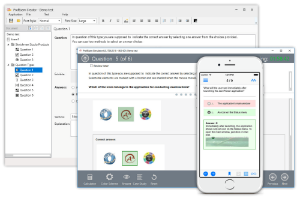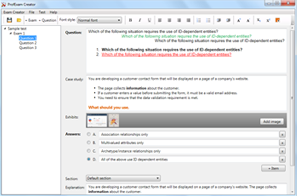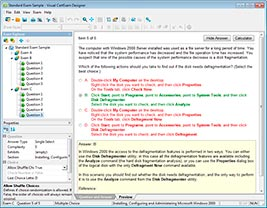## File Info

 Exam Introduction to Programming Using JavaScript Number 98-382 File Name Introduction to Programming Using JavaScript.PracticeTest.98-382.2018-05-25.1e.21q.vcex Size 1.99 Mb Posted May 25, 2018 Downloads 90

### How to open VCEX & EXAM Files?

Files with VCEX & EXAM extensions can be opened by ProfExam Simulator.

Discount: 20%

## Demo Questions

Question 1
You are creating a web page that tests a user’s ability to accurately type text. The validation should be case-insensitive.
How should you complete the code? To answer, drag appropriate functions to the correct locations. Each function may be used once, more than once, or not at all. You may need to drag the split bar between panes or scroll to view content.

Question 2
You evaluate the following code:You need to determine the values of sampleStudent, sampleCourse.name, and sampleCourse.grade that are output by console.log().
What are the final values for the three variables? To answer, select the appropriate values in the answer area.

Question 3
A JavaScript array is initialized as follows:You write the following code to manipulate the array:You need to determine the contents of the array.
Which four elements does the array contain in sequence? To answer, move the appropriate elements from the list of elements to the answer area and arrange them in the correct order.

Question 4
You need to evaluate the following code segment. Line numbers are included for reference only.Use the drop-down menus to select the answer choice that answers each question based on the information presented in the code segment.

Question 5
You are writing an engineering application. You need to create a function that will round numbers to 3 or more decimal places.
You need to create a function that receives the following two parameters:
The value parameter is the number to be formatted
The digits parameter is the number of digits to display
The function must return the value with the number of digits specified.
Which function should you use?

• A:• B:• C:• D:Question 6
You analyze the following code fragment. Line numbers are included for reference only.Question 7
You are creating a JavaScript function that returns a date the specified number of months in the future of the current date.
The function must meet the following requirements:
Accept a number that represents the number of months to add or subtract from the current date.
Return the current data adjusted by the number of months passed into the function.
How should you complete the code? To answer, select the appropriate code segments in the answer area.
NOTE: Each correct selection is worth one point.

Question 8
You work as a JavaScript developer for Adventure Works. You are writing a simple script that performs the following actions:
Declares and initializes an array
Fills the array with 10 random integers
Adds every other number starting with the first element
How should you complete the code? To answer, select the appropriate code segments in the answer area.
NOTE: Each correct selection is worth one point.

Question 9
You are writing a JavaScript program for Blue Yonder Airlines. The program stores various information about the airline’s flights.
The program has initialized the following variables:You need to determine the data type of the code segment based on initialization and the assignment of the variables. Use the drop-down menus to select the answer choice that answers each question based on the information presented in the code segment.
NOTE: Each correct selection is worth one point.

Question 10
You are planning to use the Math object in a JavaScript application. You write the following code to evaluate various Math functions:What are the final values for the three variables? To answer, select the appropriate values in the answer area.
NOTE: Each correct selection is worth one point.

CONNECT US##### PROFEXAM WITH A 20% DISCOUNT

You can buy ProfExam with a 20% discount..

HOW TO OPEN VCEX AND EXAM FILES

Use ProfExam Simulator to open VCEX and EXAM filesHOW TO OPEN VCE FILES

Use VCE Exam Simulator to open VCE files